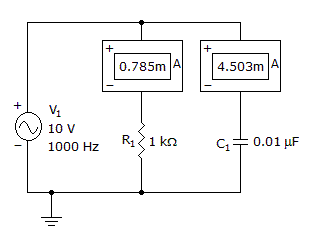# Electronics - Capacitors - Discussion

### Discussion :: Capacitors - General Questions (Q.No.14)

14.

What is the circuit's total current?[A]. 4.57 mA [B]. 9.327 mA [C]. 11.570 mA [D]. 13.830 mA

Explanation:

No answer description available for this question.

 Vasu said: (Sep 24, 2011) I= sq root (Ir^2+Ic^2) so I= sq root (0.785^2 + 4.508^2) =4.57mA

 Zohra said: (Nov 5, 2011) Not clear.

 Devadas said: (Feb 10, 2012) I=0+Ir+(11+(-11)+(0+Ic)) where 11 is a number You can take any number in that place.

 Ashutosh said: (Aug 25, 2012) Current across the capctor leads the current across the resistor by 90 in phase diagram. so,total current=sqrt(sq(Icapactor)+sq(Iresistor)).

 Ravi said: (Jan 2, 2015) We know that V=IZ where z=R//X where X=1/2PIfc.

 Anee said: (Jul 2, 2015) Current in parallel form added.

 Rishitha Chittoory said: (May 18, 2016) Why square rooting the value?

 Hirak said: (Jul 11, 2017) Why square rooting Ir & Ic?

 Jjc said: (Jul 29, 2017) Ir and Ic are not in phase (90 degrees out of phase). In a graphical analysis, they will form a right triangle, the longest side (hypotenuse) is the total current (geometric sum), which can be solved by using the Pythagorean theorem. It=sqrt(Ir^2 + Ic^2).